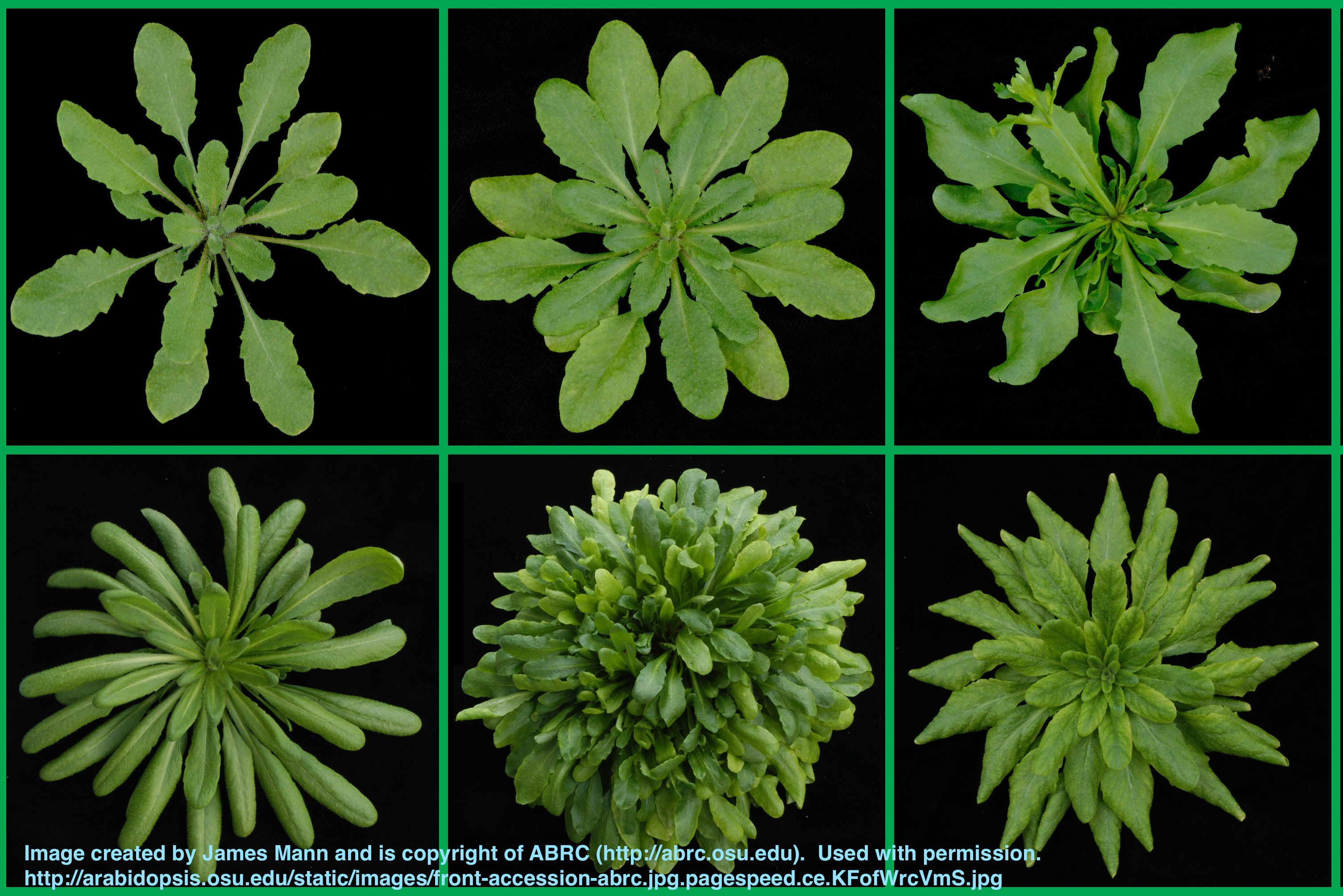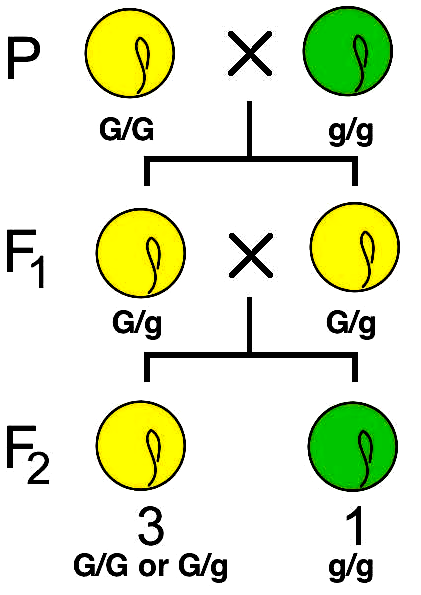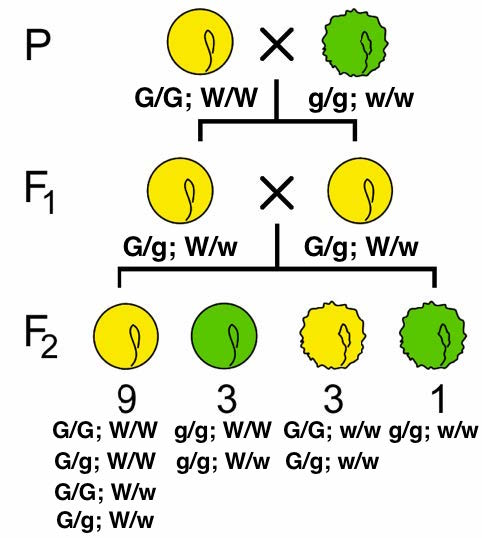# Online Open Genetics## Dihybrid Ratios2+2=4. And 3:1 + 3:1 = 9:3:3:1

Ratios and probability are among the most commonly-referenced mathematical operation for genetics. In this module, you can combine individual 3:1 ratios and predict the offspring.  The number of phenotypic classes will be 2n where n=the number of genes.  If there's only one gene, you have two phenotypes - e.g. Figure 2, Chapter 3; round vs. wrinkled seeds OR yellow vs. green.But if you combine these experiments by crossing round yellow seeds with green wrinkled ones, the results is four phenotypes (22=4). Looking at one trait each (seed shape OR seed colour) shows the underlying 3:1 ratios.

You can see in the figure to the left that there's a 3:1 ratio of round to wrinkled seeds and also a 3:1 ratio between yellow to green.

Note also that a testcross (a cross in which one parent is homozygous recessive) would show all dominant traits if the other parent is homozygous dominant, or 50% dominant traits if the other parent is heterozygous.

Ratios of 1:1, 1:0, 1:0:0:0 are possible - try out some Punnett squares of your own to investigate these.

#### Exercise: Determining Dihybrid Genotypes

In the "monohybrid" exercise, you learned to determine which trait was dominant or recessive based on the ratios of offspring. Now use the same principles to follow dihybrid ratios. More than one cross is provided. You need to:

1. Determine proper gene names based on the ratios provided.
2. Determine all possible parental genotypes. There may be more than one!

Note that "all possible" means only those that are likely to fit the ratio. While a cross between two hets could result in a 1:1 ratio instead of 3:1, it's very unlikely and should not be considered.